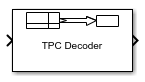# TPC Decoder

Turbo product code (TPC) decoder

Since R2018b

•Libraries:
Communications Toolbox / Error Detection and Correction / Block

## Description

The TPC Decoder block performs 2-D turbo product code (TPC) decoding of the soft input LLRs corresponding to the product code iteratively, using Chase-Pyndiah algorithm. The product code is a 2-D concatenation of linear block codes. The linear block code can be a parity check code, a Hamming code, or a BCH code capable of correcting two errors. Extended and shortened codes can be applied independently on each dimension. For a description of 2-D TPC decoding, see Turbo Product Code Decoding.

For information about valid code pairs and the error-correcting capability for each valid code pair, see Component Code Pairs.

## Ports

### Input

expand all

Log likelihood ratios, specified as a column vector.

Data Types: `double` | `single`

### Output

expand all

TPC decoded message, returned as a column vector of binary values.

Data Types: `Boolean`

Actual number of decoding iterations, returned as a positive integer.

#### Dependencies

To enable this port, select Output number of iterations executed.

Data Types: `double`

## Parameters

expand all

Row TPC parameters

Number of rows in the product code matrix, NR. The list of integer values varies depending on the setting for Extended codes.

Number of rows in the message matrix, KR. The list of integer values varies depending on the setting for Extended codes and Number of rows in code, Nr.

Select Specify shortened message length to specify a value for Number of rows in shortened message, Sr.

Number of rows in the shortened message matrix, SR, specified as an integer less than or equal to KR. When you specify this parameter, provide full-length NR and KR values to specify the (NR,KR) code pair. This code pair is then shortened to the (NRKR+SR,SR) code pair, where:

#### Dependencies

To enable this parameter, select Specify shortened message length.

Column TPC parameters

Number of columns in the product code matrix, NC. The list of integer values varies depending on the setting for Extended codes.

Number of columns in the message matrix, KC. The list of integer values varies depending on the setting for Extended codes and Number of columns in code, Nc.

Select Specify shortened message length to specify a value for Number of columns in shortened message, Sc.

Number of columns in the shortened message matrix, SC, specified as an integer. When you specify this parameter, provide full-length NC and KC values to specify the (NC,KC) code pair. This code pair is then shortened to the (NCKC+SC, SC) code pair, where:

#### Dependencies

To enable this parameter, select Specify shortened message length.

Maximum number of decoding iterations, specified as a positive integer.

Select Stop iterating when code converges to terminate decoding early if the calculated syndrome or parity-check of the component code evaluates to zero before Maximum number of iterations.

Select this parameter to add the `Iter` output port and output the actual number of TPC decoding iterations performed.

Type of simulation to run, specified as `Code generation` or `Interpreted execution`.

• `Code generation` — Simulate the model by using generated C code. The first time you run a simulation, Simulink® generates C code for the block. The model reuses the C code for subsequent simulations unless the model changes. This option requires additional startup time, but the speed of the subsequent simulations is faster than with the `Interpreted execution` option.

• `Interpreted execution` — Simulate the model by using the MATLAB® interpreter. This option shortens startup time, but the speed of subsequent simulations is slower than with the ```Code generation``` option. In this mode, you can debug the source code of the block.

## Block Characteristics

 Data Types `Boolean` | `double` | `single` Multidimensional Signals `no` Variable-Size Signals `no`